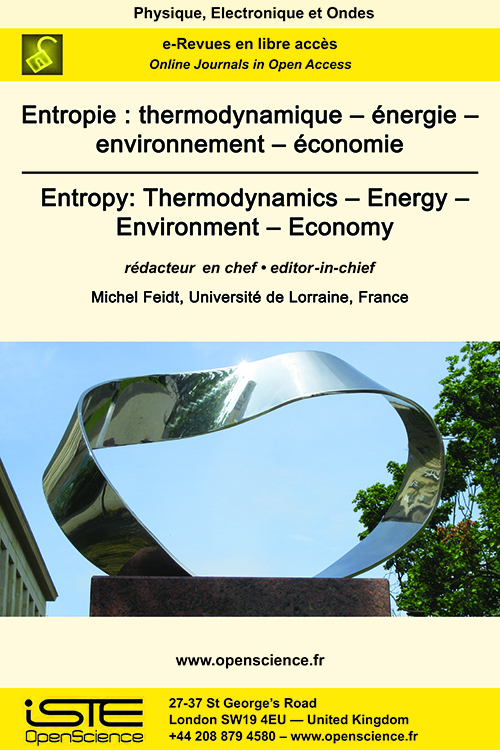# Vol 2 - Issue 1

## List of Articles

Scale-Invariant Model of Boltzmann Statistical Mechanics and Generalized Thermodynamics

Some implications of a scale-invariant model of Boltzmann statistical mechanics to the laws of generalized thermodynamics are investigated. Through definition of stochastic Planck and Boltzmann universal constants, dimension of Kelvin absolute temperature T (degrees kelvin) is identified as a length (meters) associated with Wien wavelength Tβ = λ of particle thermal oscillations. Hence, thermodynamic temperature and atomic mass of the field 𝔽β at scale β provide internal measures of (extension, duration) of background space 𝕊β+1 = 𝔽β needed to define external space and time coordinates and atomic-mass-unit of 𝔽β+1. Introduction of invariant internal thermodynamic spacetime and Boltzmann factor are in harmony with modern concepts of quantum gravity as deterministic dissipative dynamic system . The connections between de Pretto number 8338 and Joule-Mayer mechanical equivalent of heat Jc = 4.169 kJ / kcal and universal gas constant Ro = 8338 J / kcal.m are identified leading to the modified mechanical equivalent of heat J = 2Jc = 8338 J / kcal . It is shown that with work defined as Helmholtz free energy W = F = UTS, Helmholtz decomposition of total thermal energy into free heat U and latent heat pV results in modified form of the first law of thermodynamics Q = H = U- W = U + pV. Finally, by application of Boltzmann combinatorics method, entropy of ideal gas is expressed in terms of the number of Heisenberg-Kramers virtual oscillators as S = 4Nk in exact agreement with predicted entropy of black hole by Major and Setter .

Aggregative processes in open systems: simulations and detailed thermodynamic analysis by means of the IdEP-IdLA model

The IdEP-IdLA mathematical model, already used in previous works for the study of aggregative processes in closed systems, is here re-proposed for an in-depth study of some thermodynamic aspects of aggregative reactions far from equilibrium. The responses provided by the model when the system reaches the stationary state, are in line with the thermodynamics of non equilibrium: in particular, the numerical results obtained confirm the theorem of minimum entropy production, thus confirming the correctness of the theoretical approach and the reliability of the method. As a result, the model is pushed to simulate, far from equilibrium, the behaviour of dissipative structures: the codified heteropoietic aggregation is acknowledged as the proper mechanism that, within the model, reproduces the thermodynamics of these phenomena in which disorder generates order. The resulting entropic balance initiates a line of reasoning on the causes of dissipative structures.

Use of the IdEP-IdLA model for the study of aggregative processes in closed systems

As a consequence of the results obtained in a previous work, the study of aggregative reactions in closed gas systems is here addressed. Using the IdEP-IdLA mathematical model, the fundamental formulas that describe both autopoietic and heteropoietic aggregative processes are expounded. Particular emphasis is placed on the different roles that chance and necessity play according to the coding level of the processes themselves. The model responds coherently with the physical chemistry of reactions in a wide range of conditions, thus being accredited as a promising tool of theoretical investigation. In particular, in heteropoietic processes, the need to extend the entropic balance to coding agents (not only as order promoters but also as producers of compensatory entropy) is demonstrated.

Mathematical modeling for the simulation of aggregative processes

Through the use of the statistical concept of allocation entropy and by combining theoretical elements derived from statistical thermodynamics and information theory, a Markovian expression of the absolute entropy of a gaseous mixture is achieved. In the formula, the quality of the gas is represented by the entropy of a Markov source describing the mixture in terms of concentrations of single types of particles involved. The special structure of this formula opens the way for the construction of a theoretical model for the study of codified aggregative phenomena. The concepts of “ideal elementary particle” and “ideal aggregate” are firstly defined and then a particular reaction is proposed as a hypothetical aggregative process. Then, relations that describe the thermodynamics of the formation process of even very complex structures are obtained as a consequence of the combination rules. These are expressed in terms of “coding factor”, a kind of necessity rate on a random basis. In fact, thanks to the use of the Markovian expression of absolute entropy, the elaborated model allows the use of more or less stringent aggregation codes so as to simulate environments totally dominated by chance or totally deterministic, passing with continuity through all possible intermediate situations. Finally, the structure of the model permits to distinguish between processes that develop as a consequence of aggregative inclinations implicit in the system itself (autopoietic processes) and processes that develop as a consequence of the ordering action of entities outside the system (heteropoietic processes).

4D Printing: Comfort blindness and/or a systemic approach prohibited?

By adding possible temporal changes of form and/or functionality to additive manufacturing, 4D printing brings new potentials to this still expanding new field. While there is transgressive promise associated with this technology with high growth potential in academic research, targeted applications are far from being achieved. On the basis of this assessment, it appears that related fields, in a situation of possible disciplinary porosity, can, with some creativity, move away from the current highly self-centered work to try to re-invigorate 4D printing, provided that the risk-taking in interdisciplinary research is better supported. A proposal for a "roadmap" that is likely to be improved is proposed: it concerns on the one hand, a scientific axis where the 4D activity researched relies on original couplings between materials (including meta-materials) and processes, hybrid multi-material fabrications, and on the other hand, an organizational axis where interdisciplinarity and risk-taking would be privileged.2020

Volume 20- 1

Issue 1
Issue 2
Issue 3
Issue 4

2021

Volume 21- 2

Issue 1
Special issue
Issue 3

2022

Volume 22- 3

Issue 1